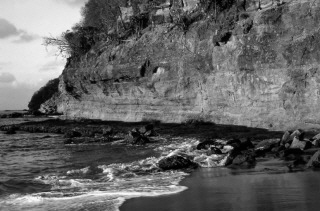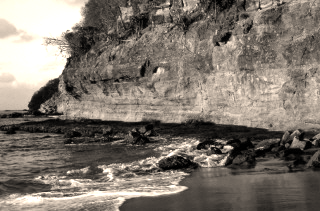﻿ ConvertToColoredGrayCommand Class | Leadtools.ImageProcessing.Color | Raster, Medical, Document Help
←Select platform
In This Topic ▼

# ConvertToColoredGrayCommand Class

Summary
Converts an image to a grayscale image, then enhances the color components based on the class properties. This class is available in the Document Toolkits.
Syntax
C#
Objective-C
C++/CLI
Java
``public class ConvertToColoredGrayCommand : RasterCommand ``
``@interface LTConvertToColoredGrayCommand : LTRasterCommand ``
````public class ConvertToColoredGrayCommand `
`    extends RasterCommand ````
``public ref class ConvertToColoredGrayCommand : public RasterCommand   ``
Remarks
• This class is more general than the GrayScaleExtendedCommand class. The ConvertToColoredGrayCommand class can be used to give images a "sepia" look by adding a blue or brown color.
• RedFactor + GreenFactor + BlueFactor must equal 1000. (Internally the values are divided by 1000).

• For each pixel in the image the red, green and blue values are modified by the RedFactor, GreenFactor and BlueFactor properties, respectively. This allows the user to give more weight to one color, essentially enhancing that color, before the pixel is converted to grayscale.

• For example, if this class is used with the RedFactor set to 500, the GreenFactor set to 250 and the BlueFactor set to 250, the red value of each pixel will get 50 percent of the weight when determining the grayscale value. The green value will get 25 percent of the weight and the blue value will get 25 percent of the weight when determining the grayscale value. This highlights or enhances the red in the image, prior to conversion to grayscale. Upon conversion to grayscale, the red, green and blue components of the output pixel are the same. Call this value G. Therefore, the RGB values for the pixel would be (G, G, G).
• Once the grayscale value for the pixel has been obtained, the red component of the output pixel can be further modified using the RedGrayFactor value. The equation for this can be seen below:
• The green and blue components are found similarly, using the GreenGrayFactor and the BlueGrayFactor values, respectively.
• Please note that the RedGrayFactor, GreenGrayFactor and BlueGrayFactor values can be negative. A negative value results in a decrease in pixel intensity. A positive value results in an increase in pixel intensity.
• If RedGrayFactor = GreenGrayFactor = BlueGrayFactor = 0, then the output is the same as the output from the GrayScaleExtendedCommand method.
• This class supports 12 and 16-bit grayscale and 48 and 64-bit color images. Support for 12 and 16-bit grayscale and 48 and 64-bit color images is available only in the Document/Medical toolkits.
• This class does not support signed data images.
• This command does not support 32-bit grayscale images.

Convert to Colored Gray Function - BeforeConvert to Colored Gray Function - AfterView additional platform support for this Convert to Colored Gray function.

Example

Run the ConvertToColoredGrayCommand on an image with RedFactor = 300, GreenFactor = 590, BlueFactor = 110, RedGrayFactor = 500, GreenGrayFactor = 300, BlueGrayFactor = 200.

C#
````using Leadtools; `
`using Leadtools.Codecs; `
`using Leadtools.ImageProcessing.Color; `
` `
` `
`public void ConvertToColoredGrayCommandExample() `
`{ `
`   // Load an image `
`   RasterCodecs codecs = new RasterCodecs(); `
`   codecs.ThrowExceptionsOnInvalidImages = true; `
` `
`   RasterImage image = codecs.Load(Path.Combine(LEAD_VARS.ImagesDir, "Master.jpg")); `
` `
`   // Prepare the command `
`   ConvertToColoredGrayCommand command = new ConvertToColoredGrayCommand(); `
`   //These arguments will transform the image into a grayscale image. `
`   command.RedFactor = 300; `
`   command.GreenFactor = 590; `
`   command.BlueFactor = 110; `
`   command.RedGrayFactor = 500; `
`   command.GreenGrayFactor = 300; `
`   command.BlueGrayFactor = 200; `
`   command.Run(image); `
`   codecs.Save(image, Path.Combine(LEAD_VARS.ImagesDir, "Result.jpg"), RasterImageFormat.Jpeg, 24); `
` `
`} `
` `
`static class LEAD_VARS `
`{ `
`   public const string ImagesDir = @"C:\LEADTOOLS22\Resources\Images"; `
`} ````
Requirements
Help Version 22.0.2023.1.25